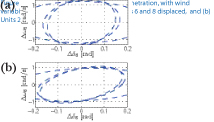Christine Chen with advisor A. Domínguez-García

There exists a need for new tools to assess the impact of renewable resource integration on power system dynamic performance, due to the highly variable and unpredictable nature of renewable power generation. Our tool provides worst-case estimates on deviations in system variables of interest, such as machine speed and system frequency. These estimates, or reach sets, are computed from short-term wind speed and solar insolation forecasts, in conjunction with a linearized power system model. The general framework is illustrated in Figure 40. Previously, we illustrated the method with a single-machine inﬁnite-bus system example, the simpliﬁed Western Electricity Coordinating Council (WECC) system.Figure 41: NE system with 30% renewable penetration, with wind variability of ±5m/s. of units 2 and 4: (a) Units 6 and 8 displaced, and (b) Units 2 and 4 displaced.

Here, we apply the reachability analysis to the 39-bus New England system model, which contains 10 synchronous units. To mimic the effect of increased renewable penetration, we replace existing conventional units with wind resources and model them accordingly. Some results are shown in Figure 41. Each dashed trace represents a set that bounds the reach set of the linearized system. So the exact reach set of the linearized system is closely upper-bounded by the intersection of all sets shown in dashed traces. Both graphs in Figure 41 illustrate variations in the machine angle and speed for unit 5, where 20% of the conventional power generation is replaced with wind power, with short-term wind speed variability of ±5 m/s. In Figure 41(a), the deviations shown are for the case where units 6 and 8 are displaced, whereas in Figure 41(b), it is shown for displacement of units 2 and 4.

This research is supported by National Science Foundation grant ECCS-0925754.